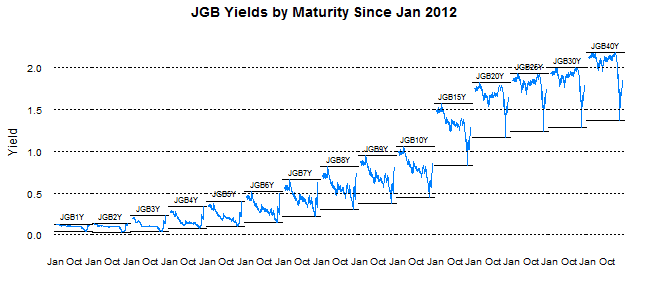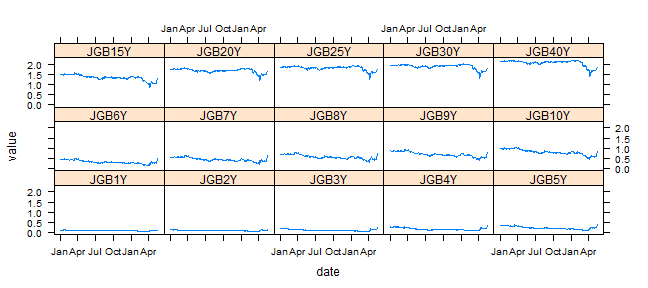Want to share your content on R-bloggers? click here if you have a blog, or here if you don't.

This blog is littered with posts about Japan. In one sentence, I think Japan presents opportunity and is a very interesting real-time test of much of my macro thinking. Proper visualization is absolutely essential for me to understand all of the dynamics. The R packages lattice and the new rCharts give me the power to see. I thought some of my recent lattice charts might help or interest some folks.

### Get and Transform the Data

```# get Japan yield data from the Ministry of
# Finance Japan data goes back to 1974

require(xts)
# require(clickme)
require(latticeExtra)

url <- "http://www.mof.go.jp/english/jgbs/reference/interest_rate/"
filenames <- paste("jgbcme", c("", "_2010", "_2000-2009",
"_1990-1999", "_1980-1989", "_1974-1979"), ".csv",
sep = "")

# load all data and combine into one jgb
# data.frame
jgb <- read.csv(paste(url, filenames, sep = ""),
stringsAsFactors = FALSE)
for (i in 2:length(filenames)) {
filenames[i], sep = ""), stringsAsFactors = FALSE))
}

# now clean up the jgb data.frame to make a jgb
# xts
jgb.xts <- as.xts(data.matrix(jgb[, 2:NCOL(jgb)]),
order.by = as.Date(jgb[, 1]))
colnames(jgb.xts) <- paste0(gsub("X", "JGB", colnames(jgb.xts)),
"Y")

# get Yen from the Fed
# getSymbols('DEXJPUS',src='FRED')

xtsMelt <- function(data) {
require(reshape2)

# translate xts to time series to json with date
# and data for this behavior will be more generic
# than the original data will not be transformed,
# so template.rmd will be changed to reflect

# convert to data frame
data.df <- data.frame(cbind(format(index(data),
"%Y-%m-%d"), coredata(data)))
colnames(data.df) = "date"
data.melt <- melt(data.df, id.vars = 1, stringsAsFactors = FALSE)
colnames(data.melt) <- c("date", "indexname", "value")
# remove periods from indexnames to prevent
# javascript confusion these . usually come from
# spaces in the colnames when melted
data.melt[, "indexname"] <- apply(matrix(data.melt[,
"indexname"]), 2, gsub, pattern = "[.]", replacement = "")
return(data.melt)
# return(df2json(na.omit(data.melt)))

}

jgb.melt <- xtsMelt(jgb.xts["2012::", ])
jgb.melt\$date <- as.Date(jgb.melt\$date)
jgb.melt\$value <- as.numeric(jgb.melt\$value)
jgb.melt\$indexname <- factor(jgb.melt\$indexname, levels = colnames(jgb.xts))
```

### Favorite Plot - Time Series Line of JGB Yields by Maturity

```p2 <- xyplot(value ~ date | indexname, data = jgb.melt,
type = "l", layout = c(length(unique(jgb.melt\$indexname)),
1), panel = function(x, y, ...) {
panel.abline(h = c(min(y), max(y)))
panel.xyplot(x = x, y = y, ...)
panel.text(x = x[length(x)/2], y = max(y),
labels = levels(jgb.melt\$indexname)[panel.number()],
cex = 0.7, pos = 3)
}, scales = list(x = list(tck = c(1, 0), alternating = 1),
y = list(tck = c(1, 0), lwd = c(0, 1))), strip = FALSE,
par.settings = list(axis.line = list(col = 0)),
xlab = NULL, ylab = "Yield", main = "JGB Yields by Maturity Since Jan 2012")
p2 + layer(panel.abline(h = pretty(jgb.melt\$value),
lty = 3))
```From TimelyPortfolio

### Good Chart but Not a Favorite

As you can tell, I did not spend a lot of time formatting this one.

```p1 <- xyplot(value ~ date | indexname, data = jgb.melt,
type = "l")
p1
```From TimelyPortfolio

### Another Favorite - Yield Curve Evolution with Opacity Color Scale

```# add alpha to colors
addalpha <- function(alpha = 180, cols) {
rgbcomp <- col2rgb(cols)
rgbcomp <- alpha
return(rgb(rgbcomp, rgbcomp, rgbcomp,
rgbcomp, maxColorValue = 255))
}

p3 <- xyplot(value ~ indexname, group = date, data = jgb.melt,
type = "l", lwd = 2, col = sapply(255/(as.numeric(Sys.Date() -
jgb.melt\$date) + 1), FUN = addalpha, cols = brewer.pal("Blues",
n = 9)), main = "JGB Yield Curve Evolution Since Jan 2012")

update(asTheEconomist(p3), scales = list(x = list(cex = 0.7))) +
layer(panel.text(x = length(levels(jgb.melt\$indexname)),
y = 0.15, label = "source: Japanese Ministry of Finance",
col = "gray70", font = 3, cex = 0.8, adj = 1))
```From TimelyPortfolio

code at Gist